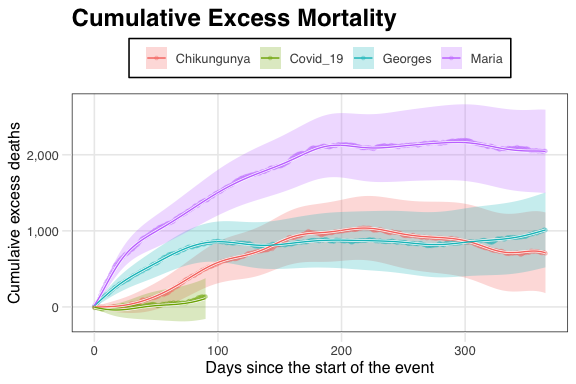Introduction to excessmort

2021-10-08

This document is an introduction to the excessmort package for analyzing time series count data. The packages was designed to help estimate excess mortality from weekly or daily death count data, but can be applied to outcomes other than death.

Data types

There are two main data types that the package works with:

• records - Each row represents a death and includes individual level information.
• count tables - Each row represents a date and includes a count and population size. These can be weekly or daily.

If you start with record-level data, it is useful to also have a data frame with population sizes for groups of interest. The pacakge functions expect a population size estimate for each date.

Record-level data

As an example of record-level data we include the cook-records dataset.

library(knitr)
library(dplyr)
library(ggplot2)
library(lubridate)
library(excessmort)
data("cook_records")
kable(cook_records[1:6,])
sex age race residenceplace date cause_1 type_of_death
male 57 white Chicago 2014-08-11 NA NA
male 78 white Forest Park 2014-08-11 Complications Of Closed Head Injury Accident
female 87 white Oak Lawn 2014-08-11 Subdural Hematoma Accident
male 26 black Chicago 2014-08-11 Multiple Gunshot Wounds Homicide
male 64 white Chicago 2014-08-11 Gunshot Wound Of The Head Suicide
male 54 white Chicago 2014-08-11 Hypertensive Cardiovascular Disease Natural

Note that this also loads a demographic data table:

# -- Cook County demographic information
kable(cook_demographics[1:6,])
sex race agegroup date population
female asian 0-4 2014-08-11 10910
female asian 0-4 2014-08-12 10910
female asian 0-4 2014-08-13 10910
female asian 0-4 2014-08-14 10910
female asian 0-4 2014-08-15 10910
female asian 0-4 2014-08-16 10910

If you have record-level data, a first step in the analysis is to convert it to count-level data. We provide the compute_counts function to help with this:

# -- Aggregating death counts
counts <- compute_counts(cook_records)
kable(counts[1:6,])
date outcome
2014-08-11 11
2014-08-12 17
2014-08-13 15
2014-08-14 12
2014-08-15 17
2014-08-16 12

The demo argument permits you to include demographic information:

# -- Aggregating death counts and computing population size from demographic data
counts <- compute_counts(cook_records, demo = cook_demographics)
kable(counts[1:6,])
date outcome population
2014-08-11 11 5238216
2014-08-12 17 5238216
2014-08-13 15 5238216
2014-08-14 12 5238216
2014-08-15 17 5238216
2014-08-16 12 5238216

Note that the table provided to the demo argument must have population size for each date of interest. The function approx_demographics can interpolate yearly data into daily data. The function get_demographics can help you get data directly from the Census. But it uses the tidycensus package which requires a Census API. You can obtain one at http://api.census.gov/data/key_signup.html, and then supply the key to the census_api_key function to use it throughout your tidycensus session.

The compute_counts has a special argument to define agegroups which you can use like this:

# -- Aggregating death counts and computing population size by age groups
counts <- compute_counts(cook_records, by = "agegroup", demo = cook_demographics,
breaks = c(0, 20, 40, 60, 80, Inf))
kable(counts[1:6,])
date agegroup outcome population
2014-08-11 0-19 0 1301842
2014-08-11 20-39 2 1580255
2014-08-11 40-59 4 1370081
2014-08-11 60-79 4 801279
2014-08-11 80-Inf 1 184759
2014-08-12 0-19 0 1301842

The breaks need to be a subset of the breaks used in the demographic data frame. The most commonly used breaks in demographic recordsare $$0, 5, 10, 15, \dots, 85, \infty$$. You can also obtain counts for different demographics as long as they are included in the records-level data. A population size will be provided as long as the demographic variables match.

# -- Aggregating death counts and computing population size by age groups, race, and sex
counts <- compute_counts(cook_records, by = c("agegroup", "race", "sex"),
demo = cook_demographics,
breaks = c(0, 20, 40, 60, 80, Inf))
kable(counts[1:6,])
date agegroup race sex outcome population
2014-08-11 0-19 asian female 0 38986
2014-08-11 0-19 asian male 0 39911
2014-08-11 0-19 asian unknown 0 NA
2014-08-11 0-19 black female 0 161098
2014-08-11 0-19 black male 0 162955
2014-08-11 0-19 black unknown 0 NA

Count-level data

Count-level data are assumed to have at least three columns: date, outcome and population. These exact names need to be used for some of the package functions to work.

The package includes several examples of count-level data:

Dataset Description
cdc_state_counts Weekly death counts for each USA state
icd (puerto_rico_icd) Puerto Rico daily mortality by cause of death
louisiana_counts Louisiana daily mortality
new_jersey_counts New Jersey daily mortality
puerto_rico_counts Puerto Rico daily mortality
puerto_rico_icd Puerto Rico daily mortality by cause of death

Computing Expected counts

A first step in most analyses is to estimate the expected count. The compute_expected function does this. We do this by assuming the counts $$Y_t$$ are an overdispresed Poisson random variable with expected value $\begin{equation} \mu_t = N_t \exp[\alpha(t) + s(t) + w(t)] \end{equation}$ with $$N_t$$ the population at time $$t$$, $$\alpha(t)$$ a slow trend to account for the increase in life expectancy we have seen in the last few decades, a seasonal trend $$s(t)$$ to account for more deaths during the winter, and a day of the week effect $$w(t)$$. Note that for weekly data we do not need to include $$w(t)$$.

Because we are often fitting this model to estimate the effect of a natural disaster or outbreak, we exclude dates with special events when estimating these parameters.

As an example, here we fit this model to Massachusetts weekly data from 2017 to 2020. We exclude the 2018 flu season and the 2020 COVID-19 pandemic.

# -- Dates to exclude when fitting the mean model
exclude_dates <- c(seq(make_date(2017, 12, 16), make_date(2018, 1, 16), by = "day"),
seq(make_date(2020, 1, 1), max(cdc_state_counts$date), by = "day")) The compute_expected function returns another count data table but with expected counts included: # -- Fitting mean model to data from Massachusetts counts <- cdc_state_counts %>% filter(state == "Massachusetts") %>% compute_expected(exclude = exclude_dates) ## No frequency provided, determined to be 52 measurements per year. ## Overall death rate is 8.99. kable(counts[1:6,]) state date outcome outcome_unweighted population log_expected_se expected excluded Massachusetts 2017-01-14 1310 1310 6843136 0.008 1265 FALSE Massachusetts 2017-01-21 1282 1282 6843830 0.008 1269 FALSE Massachusetts 2017-01-28 1239 1239 6844524 0.008 1271 FALSE Massachusetts 2017-02-04 1294 1294 6845217 0.007 1270 FALSE Massachusetts 2017-02-11 1262 1262 6845911 0.007 1266 FALSE Massachusetts 2017-02-18 1378 1378 6846605 0.007 1260 FALSE You can make a quick plot showing the expected and observed data using the expected_plot function: # -- Visualizing weekly counts and expected counts in blue expected_plot(counts, title = "Weekly Mortality Counts in MA")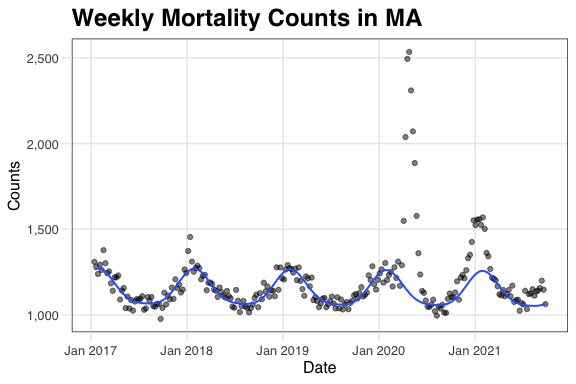You can clearly see the effects of the COVID-19 epidemic. The dispersion parameter is saved as an attribute: # -- Dispersion parameter from the mean model attr(counts, "dispersion") ##  1.36 If you want to see the estimated components of the mean model you can use the keep.components argument: # -- Fitting mean model to data from Massachusetts and retaining mean model componentss res <- cdc_state_counts %>% filter(state == "Massachusetts") %>% compute_expected(exclude = exclude_dates, keep.components = TRUE) ## No frequency provided, determined to be 52 measurements per year. ## Overall death rate is 8.99. Then, you can explore the trend and seasonal component with the expected_diagnostic function: # -- Creating diagnostic plots mean_diag <- expected_diagnostic(res) # -- Trend component mean_diag$trend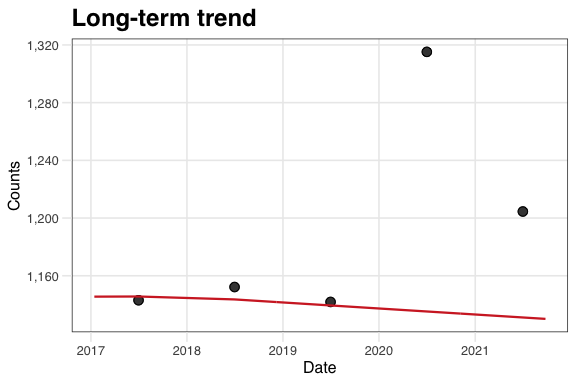# -- Seasonal component
mean_diag$seasonal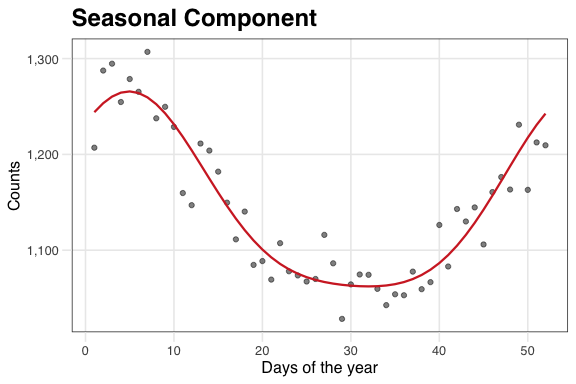Computing event effects Once we have estimated $$\mu(t)$$ we can proceed to fit a model that accounts for natural disasters or outbreaks: $Y_t \mid \varepsilon_t \sim \mbox{Poisson}\left\{ \mu_t \right[1 + f(t) \left] \varepsilon_t \right\} \mbox{ for } t = 1, \dots,T$ with $$T$$ the total number of observations, $$\mu_t$$ the expected number of deaths at time $$t$$ for a typical year, $$100 \times f(t)$$ the percent increase at time $$t$$ due to an unusual event, and $$\varepsilon_t$$ a time series of, possibly auto-correlated, random variables representing natural variability. The function excess_model fits this. We can supply the output compute_expected or we can start directly from the count table and the expected counts will be computed: # -- Fitting excess model to data from Massachusetts fit <- cdc_state_counts %>% filter(state == "Massachusetts") %>% excess_model(exclude = exclude_dates, start = min(.$date),
end = max(.$date), knots.per.year = 12, verbose = FALSE) The start and end arguments determine what dates the model is fit to. We can quickly see the results using # -- Visualizing deviations from expected mortality in Massachusetts excess_plot(fit, title = "Deviations from Expected Mortality in MA")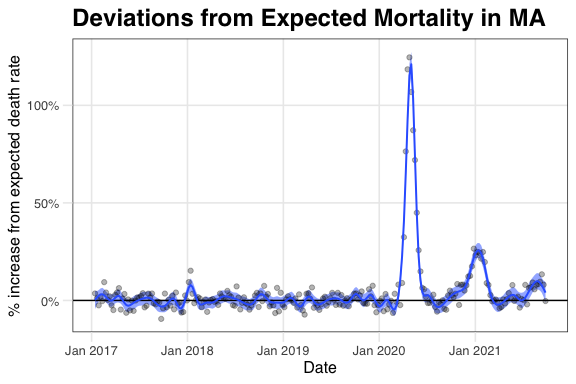The function returns dates in which a above normal rate was estimated: # -- Intervals of inordinate mortality found by the excess model fit$detected_intervals
##        start        end obs_death_rate exp_death_rate sd_death_rate observed
## 1 2017-12-30 2018-01-27           10.0           9.54        0.1201     6636
## 2 2020-03-21 2020-06-13           13.1           8.47        0.0701    22657
## 3 2020-10-10 2021-02-20           10.2           8.96        0.0581    27042
## 4 2021-07-24 2021-09-18            8.6           7.94        0.0814    10294
##   expected excess    sd fitted    se
## 1     6301    335  79.4    373  72.5
## 2    14616   8041 120.9   8174  96.1
## 3    23815   3227 154.3   3240 141.4
## 4     9497    797  97.5    743  89.6

We can also compute cumulative deaths from this fit:

# -- Computing excess deaths in Massachusetts from March 1, 2020 to May 9, 2020
cumulative_deaths  <- excess_cumulative(fit,
start = make_date(2020, 03, 01),
end   = make_date(2020, 05, 09))

# -- Visualizing cumulative excess deaths in MA
cumulative_deaths %>%
ggplot(aes(date)) +
geom_ribbon(aes(ymin = observed- 2*sd, ymax = observed + 2*sd), alpha = 0.5) +
geom_line(aes(y = observed),
color = "white",
size  = 1) +
geom_line(aes(y = observed)) +
geom_point(aes(y = observed)) +
scale_y_continuous(labels = scales::comma) +
labs(x        = "Date",
y        = "Cumulative excess deaths",
title    = "Cumulative Excess Deaths in MA",
subtitle = "During the first wave of Covid-19")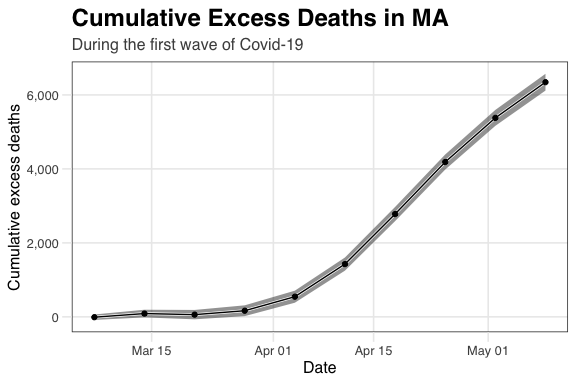We can also use this function to obtain excess deaths for specific intervals by supplying intervals instead of start and end

# -- Intervals of interest
intervals <- list(flu = seq(make_date(2017, 12, 16), make_date(2018, 2, 10), by = "day"),
covid19 = seq(make_date(2020, 03, 14), max(cdc_state_counts\$date), by = "day"))

# -- Getting excess death statistics from the excess models for the intervals of interest
cdc_state_counts %>%
filter(state == "Massachusetts") %>%
excess_model(exclude        = exclude_dates,
interval       = intervals,
verbose        = FALSE)
##              start        end obs_death_rate exp_death_rate sd_death_rate
## flu     2017-12-16 2018-02-10           9.76           9.49        0.1044
## covid19 2020-03-14 2021-09-25           9.58           8.43        0.0327
##         observed expected excess  sd
## flu        11610    11290    320 124
## covid19   103019    90746  12273 352

Daily data

With daily data we recommend using a model that accounts for correlated data. You can do this by setting the model argument to "correlated". We recommend exploring the data to see if a day of the week effect is needed and if it is included with the argument weekday.effect = TRUE.

To fit this model we need a contiguous interval of dates with $$f=0$$ to estimate the correlation structure. This interval should not be too big (default limit is 5,000 data points) as it will slow down the estimation procedure.

We demonstrate this with data from Puerto Rico. These data are provided for each age group:

data("puerto_rico_counts")
## # A tibble: 6 x 5
##   date       agegroup sex    outcome population
##   <date>     <fct>    <chr>    <dbl>      <dbl>
## 1 1985-01-01 0-4      female       1    159829.
## 2 1985-01-01 0-4      male         1    164995.
## 3 1985-01-01 5-9      female       1    160248.
## 4 1985-01-01 5-9      male         0    166447.
## 5 1985-01-01 10-14    female       0    168104.
## 6 1985-01-01 10-14    male         1    174405.

We start by collapsing the dataset into bigger agegroups using the collapse_counts_by_age functions:

# -- Aggregating data by age groups
counts <- collapse_counts_by_age(puerto_rico_counts,
breaks = c(0, 5, 20, 40, 60, 75, Inf)) %>%
group_by(date, agegroup) %>%
summarize(population = sum(population),
outcome    = sum(outcome)) %>%
ungroup()
## summarise() has grouped output by 'date'. You can override using the .groups argument.

In this example we will only use the oldest agegroup:

# -- Subsetting data; only using the data from the oldest group
counts <- filter(counts, agegroup == "75-Inf")

To fit the model we will exclude several dates due to hurricanes, dubious looking data, and the Chikungunya epidemic:

# -- Hurricane dates and dates to exclude when fitting models
hurricane_dates        <- as.Date(c("1989-09-18","1998-09-21","2017-09-20"))
hurricane_effect_ends  <- as.Date(c("1990-03-18","1999-03-21","2018-03-20"))
names(hurricane_dates) <- c("Hugo", "Georges", "Maria")
exclude_dates <- c(seq(hurricane_dates, hurricane_effect_ends, by = "day"),
seq(hurricane_dates, hurricane_effect_ends, by = "day"),
seq(hurricane_dates, hurricane_effect_ends, by = "day"),
seq(as.Date("2014-09-01"), as.Date("2015-03-21"), by = "day"),
seq(as.Date("2001-01-01"), as.Date("2001-01-15"), by = "day"),
seq(as.Date("2020-01-01"), lubridate::today(), by = "day"))

We pick the following dates to estimate the correlation function:

# -- Dates to be used for estimation of the correlated errors
control_dates <- seq(as.Date("2002-01-01"), as.Date("2013-12-31"), by = "day")

We are now ready to fit the model. We do this for 4 intervals of interest:

# -- Denoting intervals of interest
interval_start <- c(hurricane_dates,
hurricane_dates,
Chikungunya = make_date(2014, 8, 1),
Covid_19    = make_date(2020, 1, 1))

# -- Days before and after the events of interest
before <-c(365, 365, 365, 548)
after <-c(365, 365, 365, 90)

For this model we can include a discontinuity which we do for the hurricanes:

# -- Indicating wheter or not to induce a discontinuity in the model fit
disc <- c(TRUE, TRUE, FALSE, FALSE)

We can fit the model to these 4 intervals as follows:

# -- Fitting the excess model
f <- lapply(seq_along(interval_start), function(i){
excess_model(counts,
event = interval_start[i],
start = interval_start[i] - before[i],
end = interval_start[i] + after[i],
exclude = exclude_dates,
weekday.effect = TRUE,
control.dates = control_dates,
knots.per.year = 12,
discontinuity = disc[i],
model = "correlated")
})
## Computing expected counts.
## No frequency provided, determined to be 365 measurements per year.
## Overall death rate is 72.
## Order selected for AR model is 14. Estimated residual standard error is 0.053.
## Computing expected counts.
## No frequency provided, determined to be 365 measurements per year.
## Overall death rate is 72.
## Order selected for AR model is 14. Estimated residual standard error is 0.053.
## Computing expected counts.
## No frequency provided, determined to be 365 measurements per year.
## Overall death rate is 72.
## Order selected for AR model is 14. Estimated residual standard error is 0.053.
## Computing expected counts.
## No frequency provided, determined to be 365 measurements per year.
## Overall death rate is 72.
## Order selected for AR model is 14. Estimated residual standard error is 0.053.

We can examine the different hurricane effects.

This is Maria:

# -- Visualizing deviations in mortality for Hurricane Maria
excess_plot(f[],  title = names(interval_start))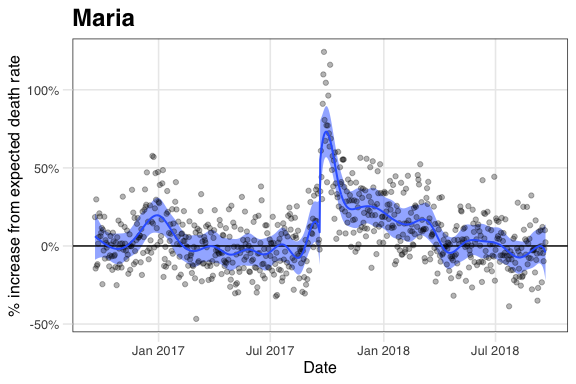You can also see the results for Georges, Chikungunya, and COVID-19 affected periods with the following code (graphs not shown to keep vignette size small)":

excess_plot(f[], title = names(interval_start))
excess_plot(f[],  title = names(interval_start))
excess_plot(f[],  title = names(interval_start))

We can compare cumulative deaths like this:

# -- Calculating excess deaths for 365 days after the start of each event
ndays <- 365
cumu <- lapply(seq_along(interval_start), function(i){
excess_cumulative(f[[i]],
start = interval_start[i],
end = pmin(make_date(2020, 3, 31), interval_start[i] + ndays)) %>%
mutate(event_day = interval_start[i], event = names(interval_start)[i])
})
cumu <- do.call(rbind, cumu)

# -- Visualizing cumulative excess deaths
cumu %>%
mutate(day = as.numeric(date - event_day)) %>%
ggplot(aes(color = event,
fill  = event)) +
geom_ribbon(aes(x    = day,
ymin = fitted - 2*se,
ymax = fitted + 2*se),
alpha = 0.25,
color = NA) +
geom_point(aes(day, observed),
alpha = 0.25,
size  = 1) +
geom_line(aes(day, fitted, group = event),
color = "white",
size  = 1) +
geom_line(aes(day, fitted)) +
scale_y_continuous(labels = scales::comma) +
labs(x     = "Days since the start of the event",
y     = "Cumulaive excess deaths",
title = "Cumulative Excess Mortality",
color = "",
fill  = "")